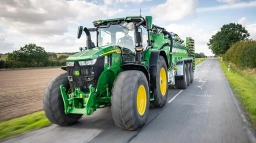# Single-digit 80674

There are cows, ducks, and geese on the farm. They have a total of 126 legs. Ducks are 3 more than houses. And there are 20 cows. If we count all the animals, we have to divide them by the number three, and we should get a single-digit number.

a =  13
b =  10
n =  43

### Step-by-step explanation:Did you find an error or inaccuracy? Feel free to write us. Thank you!

Tips for related online calculators
Do you have a linear equation or system of equations and looking for its solution? Or do you have a quadratic equation?